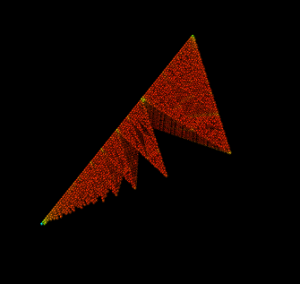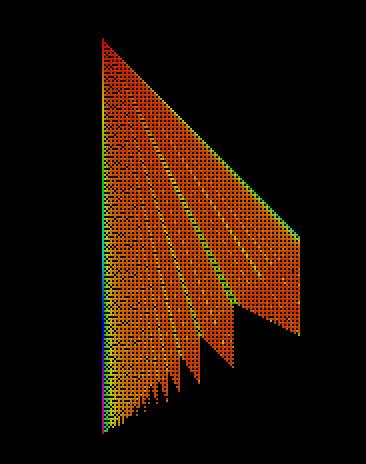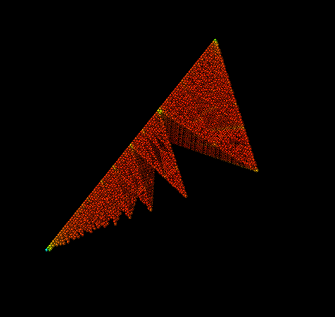# Visualizing solutions to the Markoff-Hurwitz equation

Date started: Fall 2019
Leads: Arthur Baragar and Roger Antonsen

###Abstract

The number of positive integer solutions to the Markoff-Hurwitz equation$w^2+x^2+y^2+z^2=4wxyz$ grows asymptotically like$(\log B)^{\beta+o(1)}$, where$2.43<\beta < 2.48$ (see references below). The exponent$\beta$ looks like the Hausdorff dimension of some fractal. Does it have a picture?

We consider the Euclid-like tree generated by the branches$(a,b,c)$ maps to$(a,a+b,a+c)$,$(b,b+a,b+c)$ and$(c,c+a,c+b)$ and rooted at$(1,1,2)$, and plot those points. Modulo scaling, this appears to be the fractal.

### Media

Roger Antonsen programed this into Processing (Java).The object appears to be a cone whose cross sections is an infinite sequence of Serpinski triangles.  So we also looked at a cross section:Gamburd, Magee, and Ronan also observed this sequence of triangles by noting what space is not covered after iterations of the generating maps. See their Figure 2.

However, the cone must also have some thinness, as the dimension$\beta$ is less than one plus the Hausdorff dimension of the Serpinski triangle.

### References

• Baragar, Arthur. “The exponent for the Markof-Hurwitz equations,” Pacific J. Math, 182 (1998), no. 1, 1 — 21. DOI: 10.2140/pjm.1998.182.1
• Gamburd, Alex, Michael Magee, Ryan Ronan. “An asymptotic formula for integer points on Markoff-Hurwitz varieties,” Ann. of Math. (2) 190 (2019), no. 3, 751 — 809. DOI: https://doi.org/10.4007/annals.2019.190.3.2